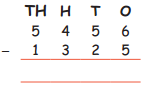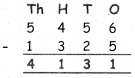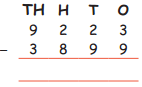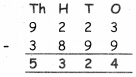Students can download 4th Maths Term 1 Chapter 2 Numbers InText Questions and Answers, Notes, Samacheer Kalvi 4th Maths Guide Pdf helps you to revise the complete Tamilnadu State Board New Syllabus, helps students complete homework assignments and to score high marks in board exams.

## Tamilnadu Samacheer Kalvi 4th Maths Solutions Term 1 Chapter 2 Numbers InText Questions

Activity (Texk Book Page No. 22)

Circle the odd numbers and write their names in the table given below.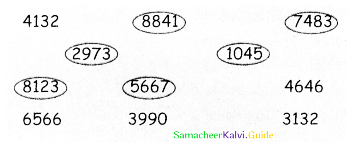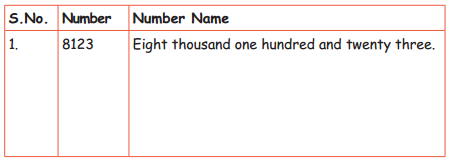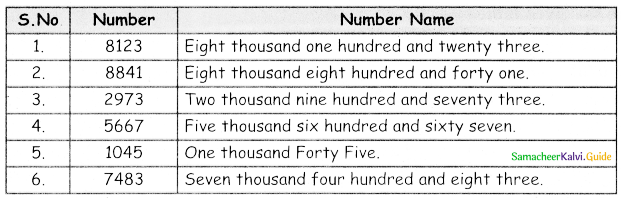Activity (Texk Book Page No. 25)

Procedure:
1. Divide the class in groups of 5.
2. Form a number using number cards from 1 to 9
3. Write its number name.
4. Write its expanded form.
5. Write the place value of each digit.
The teacher will keep checking the work done by the groups.
Activity to be done by the students themselvesTry This (Texk Book Page No. 25)

Who am I?
Who am I?
(i) Tens place is 7 = 7
(ii) Thousandth place is 4 less than 10 = 6
(iii) Hundredth place is between 3 and 5 = 4
(iv) One’s place is 2 more than 6 = 8
The number is 6478.

Activity (Texk Book Page No. 27)

Question 1.
Write the strength of ecch class in your school. Arrange them in ascending and descending orders.
Activity to be done by the students themselvesQuestion 2.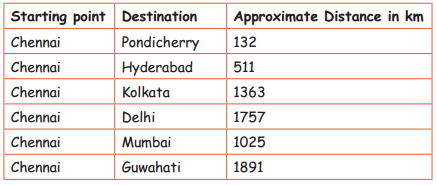Locate it in the map and find out feasible way to travel to all the places.

(a) Arrange the places in ascending order according to the distance.
Pondicherry, Hyderaibad, Mumbai, Kolkata, Delhi, Guwahati

(b) Arrange the places in descending order according to the distance.
Guwahati, Delhi, Kolkata, Mumbai, Hyderbad, Pondicherry.(c) Choose the place which is shorter in distance from Chennai.
(i) Mumbai
(ii) &uwahoti

(d) Choose the place longer in distance from Chennai.
(i) Kolkata
(ii) Mumbal
(iii) Pondicherry
(iii) Pondicherry

(e) The place in the longest distance from Chennai is ________.
Guwahati.Activity (Texk Book Page No. 33)

Write the missing numbers

(i)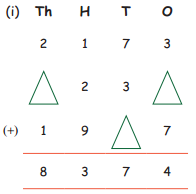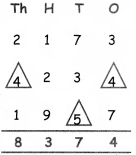(ii)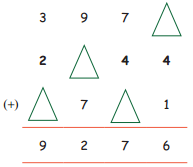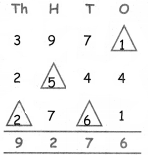Activity (Texk Book Page No. 38)

Number Puzzle:
Solve the subtraction problems. Now write the differences. One has been done for you.
(i)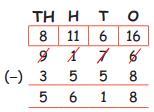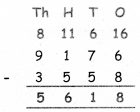(ii)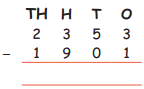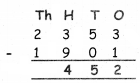(iii)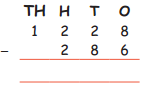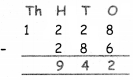(iv)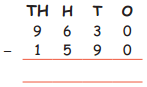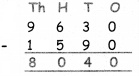(v)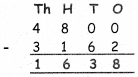(vi)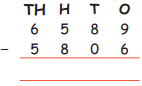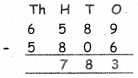(vii)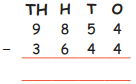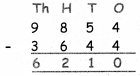(viii)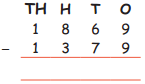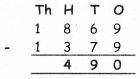(ix)